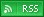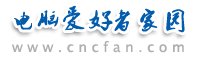电脑爱好者，提供IT资讯信息及各类编程知识文章介绍，欢迎大家来本站学习电脑知识。 最近更新 | 联系我们站内搜索：

# PHP4和PHP5对比分析：静态成员和异常处理

“对象方法” 在一个对象没有实例化前允许被调用。同样，“对象变量” 在一个对象没有实例化前可以被独立操作控制(不需要用一个对象的方法来控制)。

class calculator {
static public \$pi = 3.14151692;
static public function add(\$x,\$y) {
return \$x + \$y;
}
}
\$s = calculator::\$pi;
print（"\$result"）;
?>

class foo {
function divide(\$x,\$y) {
if(\$y==0) throw new Exception("cannot divide by zero");
return \$x/\$y;
}
}
\$x = new foo();
try {
\$x->divide(3,0);
} catch (Exception \$e) {
echo \$e->getMessage();
echo "n
n";
// Some catastrophic measure here
}
?>

 PHP热门文章排行 网站赞助商